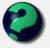# Math Is Fun Forum

Discussion about math, puzzles, games and fun.   Useful symbols: ÷ × ½ √ ∞ ≠ ≤ ≥ ≈ ⇒ ± ∈ Δ θ ∴ ∑ ∫  π  -¹ ² ³ °

You are not logged in.

## #1 2005-08-07 17:09:32

wcy
Member
Registered: 2005-08-04
Posts: 117

### Nice graphs

Do any of you have cool graphs plotted by some computer program (eg. graphmatica)

Does anyone know how to plot a star shaped graph?

Offline

## #2 2005-08-07 19:26:46

mathsyperson
ModeratorRegistered: 2005-06-22
Posts: 4,900

### Re: Nice graphs

I like graphing the sum of various sine functions in Excel. I first found out about it in Physics, when doing constructive and destructive interference of waves. If you combine about three of different frequencies and amplitudes, you can get some really nice patterns.

Last edited by mathsyperson (2005-08-09 03:08:32)

Why did the vector cross the road?
It wanted to be normal.

Offline

## #3 2005-08-07 22:50:44

NIH
MemberRegistered: 2005-06-14
Posts: 33

### Re: Nice graphs

mathsyperson wrote:

Random fact for the day: All primes of the form 4n+1 can be written as a²+b².
None of the primes of the form 4n-1 can be written in this way. (a, b, n ∈Z)
If you can prove/disprove this, you will be very rich.

Fermat proved this at the beginning of the 17th century!  In fact, he proved that all primes of the form 4n+1 can be written as the sum of two squares in exactly one way.  The proof is not trivial.  However, there is a very simple proof that no primes of the form 4n-1 can be written as the sum of two squares.

2 + 2 = 5, for large values of 2.

Offline

## #4 2005-08-08 01:33:01

mathsyperson
ModeratorRegistered: 2005-06-22
Posts: 4,900

### Re: Nice graphs

Oops! OK, fixed it. Thanks.

Why did the vector cross the road?
It wanted to be normal.

Offline

## #5 2005-08-08 19:32:09

ganeshRegistered: 2005-06-28
Posts: 40,452

### Re: Nice graphs

NIH wrote:

However, there is a very simple proof that no primes of the form 4n-1 can be written as the sum of two squares.

4n-1 = a² + b²
4n    = a² + b² + 1
n    = (a² + b² + 1)/4
Since 4n-1 is an odd number, one of a and b is odd and the other is even.
When an even number is squared, the resultant is always a multiple of 4.
An odd number when squared is always of the form 2n+1.
Any number of the form 2n+2 would never be divisible by 4 when n is even! When n is odd, it is of the form(2n+1). When this is squared, we get
4n² +4n+1. i.e. 4(n² +n)+1. When 1 is added to this number, we get
4(n² +n)+2. This number is NOT divisible by 4.
Therefore,
a² + b² ≠ 4n-1
q.e.dLast edited by ganesh (2005-08-08 20:15:03)

It appears to me that if one wants to make progress in mathematics, one should study the masters and not the pupils. - Niels Henrik Abel.

Nothing is better than reading and gaining more and more knowledge - Stephen William Hawking.

Offline

## #6 2005-08-09 01:44:02

NIH
MemberRegistered: 2005-06-14
Posts: 33

### Re: Nice graphs

A simple proof that no integer of the form 4n-1 can be written as the sum of two squares.

If n is even, say n = 2r, (2r)² = 4r² = 0 (mod 4).  (Leaves remainder 0 when divided by 4.)
If n is odd, say n = 2r + 1, (2r + 1)² = 4(r² + r) + 1 = 1 (mod 4.)

So the sum of two squares, mod 4, is always equal to 0 = 0 + 0, 1 = 0 + 1 or 1 + 0, or 2 = 1 + 1; never 3.  (Of course 4n - 1 = 3 (mod 4.))

In fact, for the square of an odd integer, we can write (2r + 1)² = 4r(r + 1) + 1.
Since one of r and r + 1 must be even, 4r(r + 1) is divisible by 8.
So the square of an odd integer leaves remainder 1 when divided by 8, which is sometimes useful.

2 + 2 = 5, for large values of 2.

Offline

## #7 2005-08-09 02:30:59

NIH
MemberRegistered: 2005-06-14
Posts: 33

### Re: Nice graphs

Try graphing y = sin(x) + sin(x°).  (y = sin(x radians) + sin(x degrees).)

Is the function periodic?

2 + 2 = 5, for large values of 2.

Offline

## #8 2005-08-09 02:59:52

mathsyperson
ModeratorRegistered: 2005-06-22
Posts: 4,900

### Re: Nice graphs

sin x° is a wave that repeats for every 360 units.
sin x is also a wave, but it repeats every 2pi units.
Adding these gives a wavy wave (hard to describe, easy to replicate in Excel)
The only way I could think of to make it a JPG was to copy and paste it into paint and save it from there, so I'm unsure of how good the quality will be, but I've uploaded it into the image gallery.

The combined function would repeat itself every (lowest common multiple of 360 and 2pi) units, but as pi is transcendental that will never happen. So, it will be a wavy wave that looks almost exactly the same for whatever range of x values spanning 360 you choose, but it will actually always be slightly different.

Last edited by mathsyperson (2005-08-09 03:13:14)

Why did the vector cross the road?
It wanted to be normal.

Offline